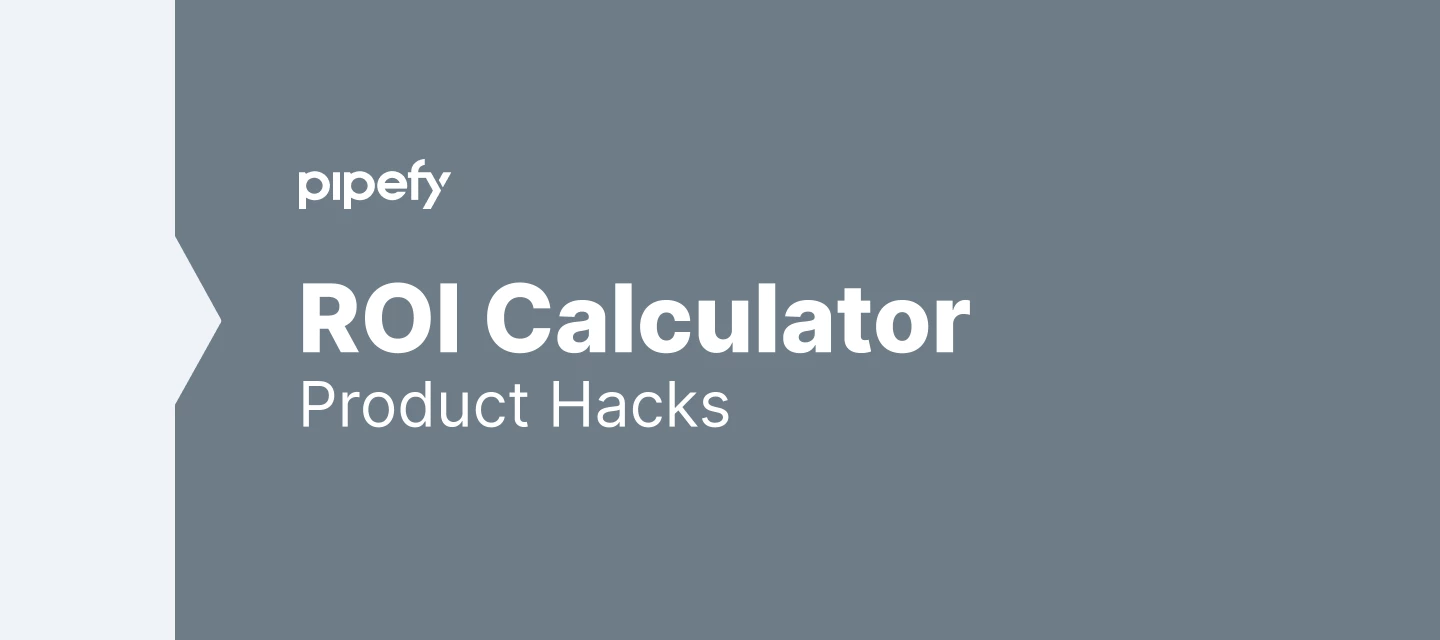PRODUCT HACKS

# How to calculate your return over investment using Pipefy?

• 0 replies
• 162 views• Rica• Pipefy Staff
• 4 replies

Do you know you can easily calculate the ROI (return on investment) on your process?

There are multiple features that save you a lot of time, like: automation rules, automatic emails, PDF generator and connected cards.

On average all the features above save up to 5 minutes of work from a Pipefy user every time they run.

To know how many times these features ran in your process, last month for example, just ask your CSM (Customer Success Manager)! They can pull this data and share it with you.

ROI, is a pretty straightforward formula: ROI = ((Gain - Investment) / Investment). The result you have  to multiply by100 to have the value on percentage (%).

Okay, but how do I calculate ROI using these features? Well, like Benjamin Franklin said: “Time is money”.

If you have how many automatic emails were sent in a month, you can calculate how many minutes you saved with this feature. Knowing the average wage of your team members (per hour) you can multiply it  by the number of hours saved to know the total amount of savings in \$\$\$.

For example:

Process A sends 300 automatic emails per month.

Each email saves 5 minutes per Pipefy User.

300 x 5 = 1.500 minutes saved in a month. 1.500 divided by 60 minutes = 25 hours saved in a month.

If the average hourly wage of this company is \$10,  25 x 10 = \$250 saved in a month with automatic  emails.

If your monthly contract with Pipefy is \$100/month.

So, ROI = (\$250 - \$100) / \$100

ROI = 1,5

If you want to see the result on percentage (%), you should multiply the result by 100.

In this case 1,5 x 100 = 150%

So the final result of the ROI in this example is 150%.

I hope that was useful :)